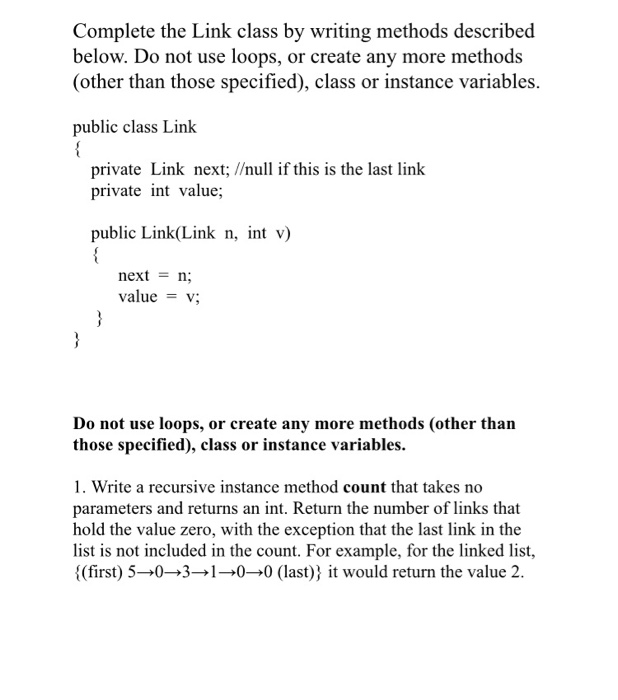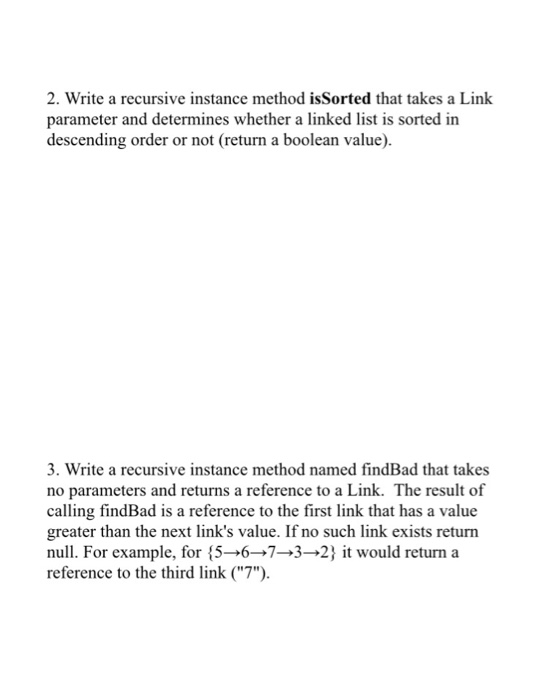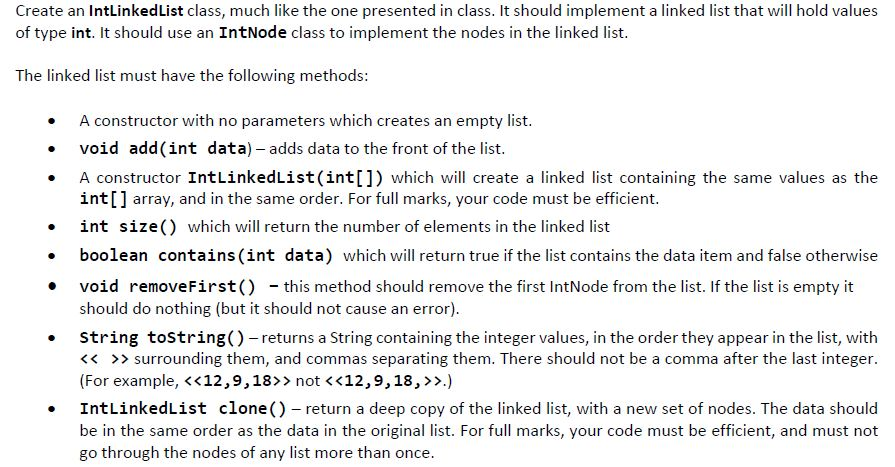# Need the answers to these RECURSIVE practice problems using Linked Lists in JAVA . Thank you. Complete the Link cl...

Need the answers to these RECURSIVE practice problems using Linked Lists in JAVA . Thank you.Complete the Link class by writing methods described below. Do not use loops, or create any more methods (other than those specified), class or instance variables. public class Link private Link next; /null if this is the last link private int value; public Link(Link n, int v) nextn valuev; Do not use loops, or create any more methods (other than those specified), class or instance variables. 1. Write a recursive instance method count that takes no parameters and returns an int. Return the number of links that hold the value zero, with the exception that the last link in the list is not included in the count. For example, for the linked list, {(first) 5→0→3→1→0→0 (last)) it would return the value 2.
2. Write a recursive instance method isSorted that takes a Link parameter and determines whether a linked list is sorted in descending order or not (retun a boolean value). 3. Write a recursive instance method named findBad that takes no parameters and returns a reference to a Link. The result of calling findBad is a reference to the first link that has a value greater than the next link's value. If no such link exists return null. For example, for {5→6→7→3→2) it would return a reference to the third link ("7").

//Java program

private int value;

next = n;
value = v;
}
return next;
}
next = n;
}
public int getValue() {
return value;
}
public void setValue(int v) {
value = v;
}
}

if(node==null||node.getNext()==null)return 0;
if(node.getValue()==0)return 1 + countRec(node.getNext());
else return countRec(node.getNext());

}
if(node==null||node.getNext()==null)return null;
if(node.getValue()>node.getNext().getValue())return node;
}

}
public void append(int data) {
return;
}
while(current.getNext()!=null)current = current.getNext();
current.setNext(node);
}

public void print() {
while(current!=null) {
System.out.print(current.getValue()+" ");
current = current.getNext();
}
System.out.println();
}

public int count() {
}

}

}

public static void main(String args[]) {
list.append(5);
list.append(6);
list.append(7);
list.append(3);
list.append(2);

}

public static boolean isSorted(Link node) {
if(node==null||node.getNext()==null)return true;
if(node.getValue()>=node.getNext().getValue())return isSorted(node.getNext());
return false;
}
}

Similar Homework Help Questions
• ### Linked list and recursion. I wrote 2 classes IntNode and IntLinkedList according to the question ...linked list and recursion. I wrote 2 classes IntNode and IntLinkedList according to the question below , but when I run the code using a test file which I'm posting below both the test file and the solution I get a nullPointerException. class IntNode { protected int data; protected IntNode link; /* Constructor */ public IntNode() { link = null; data = 0; } /* Constructor */ public IntNode(int d,IntNode n) { data = d; link = n; } /*...

• ### java

The LinkedList will be tested using strings only.Specifically, the following methods must be implemented in the LinkedList class:(You should utilize listIterator() method already defined in the LinkedList class to obtain its LinkedListIterator object, and use the methods in theLinkedListIterator class to traverse from the first element to the last element of the linked list to define the following methods.)1.public String toString()The toString method should concatenate strings in the linked list, and return a string of the following format:{ Orange Banana...

• ### Recursive java function

Suppose that you have a linked list of integers containing nodes that areinstances of the following class:public class IntNode {private int val;private IntNode next;}a. Write a method named printOddsRecursive() that takes a reference to thefirst node in a linked list of IntNodes and uses recursion to print the oddvalues in the list (if any), with each value printed on a separate line. If thereare no odd values, the method should not do any printing.b. Write a method named printOddsIterative() that...

• ### Doubly Linked List Java Help Details: First, read the DoublyLinkedList.java code and try to under...

Doubly Linked List Java Help Details: First, read the DoublyLinkedList.java code and try to understand what each field stores and what each method is doing. Modify and complete the class as described below •The field size was defined in the class but was never maintained. Set the current default value and modify it whenever it is needed in the existing methods and other methods you implement as it is needed. It should always include the number of Nodes inside the...

• ### java recursive function

public class StringNode {private char ch;private StringNode next;/*** Constructor*/public StringNode(char c, StringNode n) {ch = c;next = n;}/*** convert - converts a standard Java String object to a linked-list* string and returns a reference to the linked-list string*/public static StringNode convert(String s) {if (s.length() == 0)return null;StringNode firstNode = new StringNode(s.charAt(0), null);StringNode prevNode = firstNode;StringNode nextNode;for (int i = 1; i < s.length(); i++) {nextNode = new StringNode(s.charAt(i), null);prevNode.next = nextNode;prevNode = nextNode;}return firstNode;}public static void printEveryOther(StringNode str)This method should...

• ### Java: Linked List (Create a class, and the class needs 2 new methods)Hello!! Please help this for me!!Thank you for your time!!!!!!!!class LinkedList1{/**The Node class stores a list elementand a reference to the next node.*/private class Node{String value;Node next;/**[email protected] val The element to store in the [email protected] n The reference to the successor node.*/Node(String val, Node n){value = val;next = n;}/**[email protected] val The element to store in the node.*/Node(String val){// Call the other (sister) constructor.this(val,null);}}private Node first;// list headprivate Node last;// last element in list/**Constructor.*/public LinkedList1(){first = null;last = null;}/**The isEmpty method...

• ### Data Structures - Singly Linked Lists You will add a method swapNodes to SinglyLinkedList class (below). This method should swap two nodes node1 and node2 (and not just their contents) given reference...

Data Structures - Singly Linked Lists You will add a method swapNodes to SinglyLinkedList class (below). This method should swap two nodes node1 and node2 (and not just their contents) given references only to node1 and node2. The new method should check if node1 and node2 are the same node, etc. Write the main method to test the swapNodes method. You may need to traverse the list. package linkedlists; public class SinglyLinkedList<E> implements Cloneable {    // ---------------- nested Node class...

• ### java recursion function

public class DNode {private char ch;private DNode next;private DNode prev;}Suppose that you have a doubly-linked list (implemented using the DNode classdescribed in the above). Write a method namedremoveAllOccurrences() that takes two parameters, a reference to the firstnode of the linked list and a character ch, and removes all occurrences of ch fromthe linked list. Your method will need to return a reference to the first node inthe linked list, because the original first node may end up being removed ....

• ### Recursion help

Write a java file called RecursiveMethods and provide the following methods (thesemethods are required to be fully recursive in that they contain no loops at all):1) Write a recursive method that takes as input a String S and returns the numberof uppercase letters in S.2) Write a recursive method that finds the number of occurrences of a specifiedletter in a string using the following method header:public static int count(String str, char a)3) Write a method that checks if the first...

• ### (Creating a two-way linked list) java program

Modify the Node class to add the new field name previous to refer to the previous node in the list, as follows:public class Node{Object element;Node next;Node previous;public Node(Object o){element = o;}}Simplify the implementation of the add(Object element, int index) and remove(int index) methods to take advantage of the bidirectionallinked list.Example is a one way linked:public class MyLinkedList<E> extends MyAbstractList<E> {private Node<E> head, tail;/** Create a default list */public MyLinkedList() {}/** Create a list from an array of objects */public MyLinkedList(E[]...

Need Online Homework Help?